# Methods

We provide additional material for each method to ease the understanding and to improve your learning experience.

Fact sheet
Fact sheets summarize the main facts about a specific method
Table of content
Check the TOC of the corresponding book chapter
Excel example
Run your first multivaraite analysis with the help of Excel
R code + output
R code and output for the chocolate case study
SPSS data set
Order the SPSS data set + syntax used in the book
Lecturer support
We offer lecturer slides as well as all tables and figures
Videos
We plan to offer videos to ease the understanding of specific issues

## Basics of Data Analysis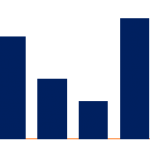The introduction serves as a refresher and reference to basic statistical measures.

## Regression Analysis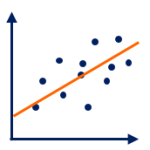Regression Analysis is the most frequently used method of multivariate analysis.

## Analysis of Variance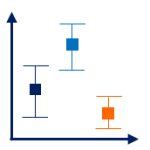Analysis of Variance (ANOVA) is frequently used to analysis experimental data.

## Discriminant Analysis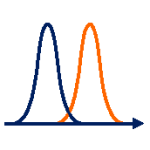Discriminant Analysis aims to identify differences across groups.

## Logistic Regression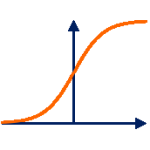Logistic regression is suited if the dependent variable is categorical.

## Contingency AnalysisContingency analysis tests whether categorical variables are associated.

## Factor AnalysisFactor analysis reduces a large number of variables to a smaller number of factors.

## Cluster Analysis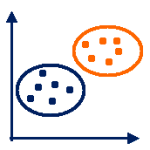Cluster analysis groups subjects or objects that are similar.